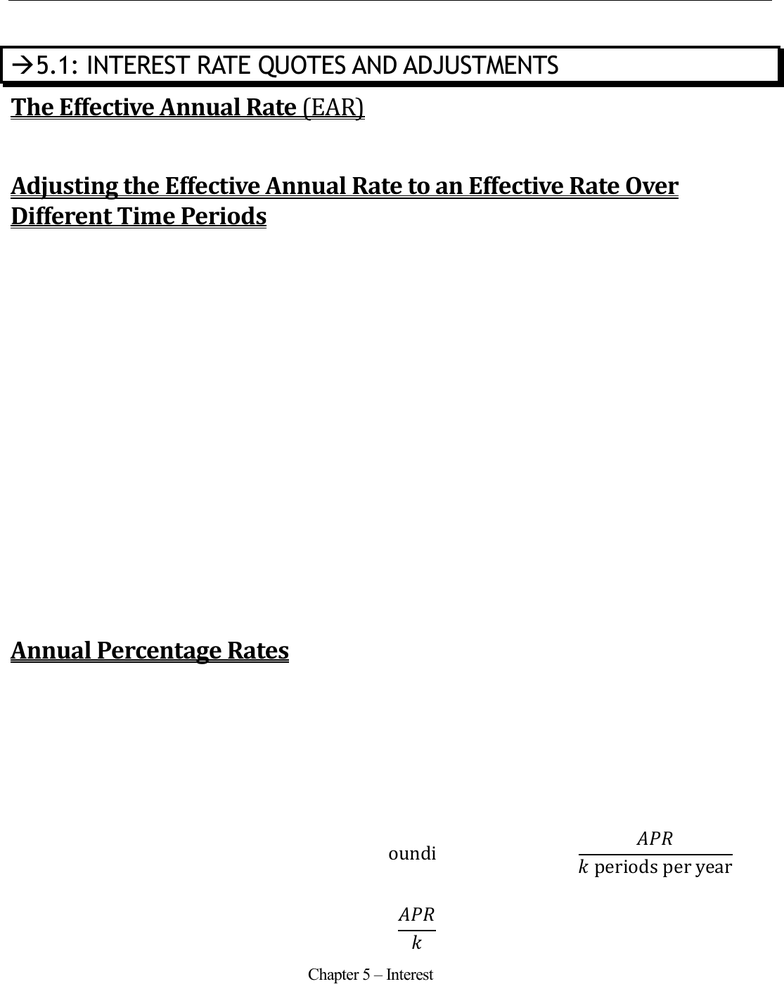Textbook Notes (280,000)
CA (160,000)
UTM (8,000)
Chapter 5

# MGT338H5 Chapter Notes - Chapter 5: Effective Interest Rate, Annual Percentage Rate, Compound Interest

Department
Management
Course Code
MGT338H5
Professor
Gabor Virag
Chapter
5

This preview shows page 1. to view the full 4 pages of the document.Prof. Gabor Virag MGT338 Page 1 of 4
Chapter 5 Interest Rates
Chapter 5 Interest Rates
Effective Annual Rates; Annual Percentage Rates; Converting APR to EAR
5.1: INTEREST RATE QUOTES AND ADJUSTMENTS
The Effective Annual Rate (EAR)
Interest rates are often stated as an effective annual rate (EAR), which indicates the total amount of
interest that will be earned at the end of one year. In Chapters 3 & 4, all interest rates were EARs.
Adjusting the Effective Annual Rate to an Effective Rate Over
Different Time Periods
Imagine an effective annual rate of 5%. A \$100,000 investment after 2 years grows to:
   
This shows that earning an effective annual rate of 5% for two years is equivalent to 10.25% in total
interest over the entire period. In general, by raising the interest rate factor (1 + r) to the appropriate
power, we can compute an equivalent effective interest rate for a longer time period.
We can use the same method to find the equivalent effective interest for periods shorter than one year.
For example, earning a 5% EAR over 6 months is equivalent to receiving:
  
A 5% EAR is equivalent to an interest rate of approximately 2.47% earned every 6 months; this
would be an effective 6-month rate.
In general, we can convert an effective rate of r for one period to an equivalent effective rate for n
periods using the following formula:
 
 
r is the original rate, and to convert it to a new rate you need to set n to the number of current-periods
you want the new period to be made up of. Ex: if r is a 6-month effective rate, and you want the
EAR, n is 2 because there are 2 6-month periods in a year.
Annual Percentage Rates
Banks quote interest rates in terms of an annual percentage rate (APR), which indicates the amount
of simple interest earned in one year. That is, the amount of interest earned without the effect of
compounding even though compounding may occur. Because it does not include the effect of
compounding, the APR is typically less than the actual amount of interest that you will earn. To get
the accurate amount of interest you will, you must convert APR to an EAR.
The APR with k compounding periods is a way of indirectly quoting the effective interest rate, r,
earned each compounding period.
   

Combining everything we know, we can convert an APR to an EAR by using the following formula:
 